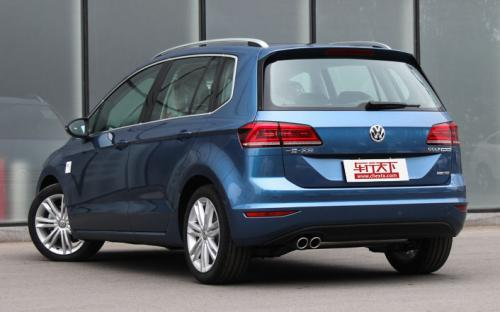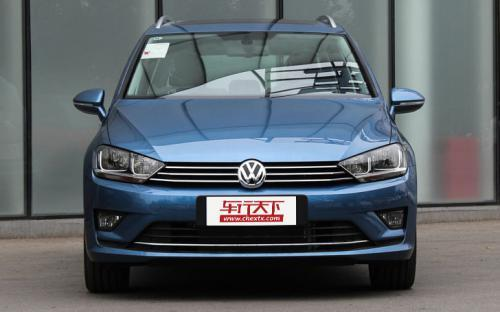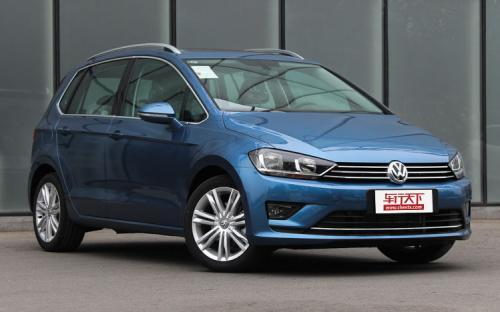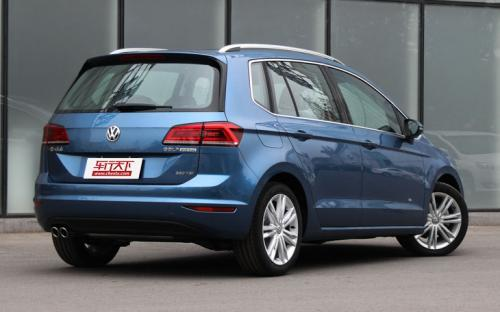## 一汽-大众 高尔夫·嘉旅9 种颜色可选2016款最低售价：13.19 万元起

4348(mm)1807(mm)1574(mm)##### 配置亮点：
• 胎压监测装置

• ISOFIX儿童座椅接口

• 车身稳定控制(ESC/ESP/DSC等)

• 电动天窗

• 定速巡航

• 后倒车雷达

• 真皮座椅

• GPS导航系统

• 氙气大灯

• 后视镜加热

2016款 230TSI 自动豪华型 (0张)
• 2016款 230TSI 自动豪华型 (0张)
• 2016款 280TSI 自动豪华型 (107张)
• 2016款 280TSI 自动旗舰型 (222张)一汽-大众 高尔夫·嘉旅 在售车型

排量 车型 厂商指导价 本地最低报价 购车工具
1.6L 1.6L 手动舒适型 5挡手动 13.19万 询底价+对比
1.6L 自动舒适型 6挡手自一体 14.39万 询底价+对比
1.2T 180TSI 自动进取型 7挡双离合 16.19万 询底价+对比
230TSI 手动进取型 5挡手动 15.29万 询底价+对比
230TSI 自动进取型 7挡双离合 16.69万 询底价+对比
230TSI 自动豪华型 7挡双离合 17.99万 询底价+对比
280TSI 自动豪华型 7挡双离合 18.49万 询底价+对比
280TSI 自动旗舰型 7挡双离合 19.79万 询底价+对比
1.4T 180TSI 自动进取型 7挡双离合 16.19万 询底价+对比
230TSI 手动进取型 5挡手动 15.29万 询底价+对比
230TSI 自动进取型 7挡双离合 16.69万 询底价+对比
230TSI 自动豪华型 7挡双离合 17.99万 询底价+对比
280TSI 自动豪华型 7挡双离合 18.49万 询底价+对比
280TSI 自动旗舰型 7挡双离合 19.79万 询底价+对比

一汽-大众 高尔夫·嘉旅 经销商

查看更多 >>
更多 >>

本地降价排名

### 一汽-大众 高尔夫·嘉旅 动力加速

高尔夫·嘉旅 0-100公里加速时间分布在 8.9-13.6秒 属于 运动级

动力级别 加速时间 车型
民用级(4款) 12.4s 1.6L 手动舒适型
13.6s 1.6L 自动舒适型
11.5s 180TSI 自动进取型
9.9s 230TSI 手动进取型230TSI 自动进取型230TSI 自动豪华型
运动级(1款) 8.9s 280TSI 自动豪华型280TSI 自动旗舰型

一汽-大众 高尔夫·嘉旅 视频

一汽-大众 高尔夫·嘉旅 新闻资讯

# 帝豪GS/新宝马X1等 5月份上市新车前瞻

即将上市 超过9202次关注

对大部分汽车媒体而言，刚结束的北京车展就是一场“噩梦”，将近200多款上市和首发新车让跑展馆的我们累得够呛。当然，对大家来说，每次的车展都是盛宴，如同世界杯...

# 多种动力选择 高尔夫·嘉旅5月6日上市

国产新车 超过8851次关注

据悉，一汽-大众高尔夫·嘉旅将于5月6日公布售价，新车延续了进口车型的造型设计，动力方面将提供多种动力选择。

猜你喜欢

﻿
• 快速找车
• 选择品牌
• 选择品牌
• A  奥迪
• A  阿斯顿·马丁
• A  阿尔法·罗密欧
• B  宝沃
• B  布加迪
• B  巴博斯
• B  保时捷
• B  宾利
• B  奔驰
• B  宝马
• B  本田
• B  别克
• B  标致
• B  比亚迪
• B  宝骏
• B  北汽制造
• B  北汽新能源
• B  北汽幻速
• B  北汽威旺
• B  北京汽车
• B  奔腾
• B  北汽绅宝
• C  长安
• C  长安商用
• C  长城
• C  昌河
• D  大众
• D  道奇
• D  DS
• D  东南
• D  东风风神
• D  东风风行
• D  东风小康
• D  东风风度
• D  东风
• F  福特
• F  丰田
• F  菲亚特
• F  法拉利
• F  福田
• F  福迪
• F  福汽启腾
• G  观致
• G  广汽传祺
• G  广汽吉奥
• G  GMC
• H  红旗
• H  汉腾汽车
• H  哈弗
• H  哈飞
• H  海格
• H  海马
• H  华颂
• H  黄海
• H  华泰
• H  恒天
• J  吉利汽车
• J  捷豹
• J  Jeep
• J  江淮
• J  江铃
• J  金杯
• J  九龙
• J  金旅
• K  凯翼
• K  凯迪拉克
• K  克莱斯勒
• K  科尼塞克
• K  卡威
• K  开瑞
• L  路虎
• L  林肯
• L  劳斯莱斯
• L  兰博基尼
• L  雷克萨斯
• L  铃木
• L  雷诺
• L  理念
• L  力帆
• L  莲花汽车
• L  猎豹
• L  路特斯
• L  陆风
• M  马自达
• M  MG
• M  MINI
• M  玛莎拉蒂
• M  摩根
• M  迈凯轮
• N  纳智捷
• O  欧宝
• O  讴歌
• O  欧朗
• Q  奇瑞
• Q  起亚
• Q  启辰
• R  日产
• R  荣威
• R  瑞麒
• S  三菱
• S  斯威汽车
• S  萨博
• S  smart
• S  斯柯达
• S  斯巴鲁
• S  思铭
• S  双龙
• S  上汽大通
• S  双环
• T  特斯拉
• T  腾势
• W  沃尔沃
• W  五菱汽车
• W  五十铃
• W  威兹曼
• W  威麟
• X  现代
• X  雪佛兰
• X  雪铁龙
• X  西雅特
• Y  一汽
• Y  英菲尼迪
• Y  英致
• Y  依维柯
• Y  野马汽车
• Y  永源
• Z  众泰
• Z  中华
• Z  中兴
• Z  知豆
• 选择车系
• 选择车系
• 车型对比
• 选择品牌
• 选择品牌
• A  奥迪
• A  阿斯顿·马丁
• A  阿尔法·罗密欧
• B  宝沃
• B  布加迪
• B  巴博斯
• B  保时捷
• B  宾利
• B  奔驰
• B  宝马
• B  本田
• B  别克
• B  标致
• B  比亚迪
• B  宝骏
• B  北汽制造
• B  北汽新能源
• B  北汽幻速
• B  北汽威旺
• B  北京汽车
• B  奔腾
• B  北汽绅宝
• C  长安
• C  长安商用
• C  长城
• C  昌河
• D  大众
• D  道奇
• D  DS
• D  东南
• D  东风风神
• D  东风风行
• D  东风小康
• D  东风风度
• D  东风
• F  福特
• F  丰田
• F  菲亚特
• F  法拉利
• F  福田
• F  福迪
• F  福汽启腾
• G  观致
• G  广汽传祺
• G  广汽吉奥
• G  GMC
• H  红旗
• H  汉腾汽车
• H  哈弗
• H  哈飞
• H  海格
• H  海马
• H  华颂
• H  黄海
• H  华泰
• H  恒天
• J  吉利汽车
• J  捷豹
• J  Jeep
• J  江淮
• J  江铃
• J  金杯
• J  九龙
• J  金旅
• K  凯翼
• K  凯迪拉克
• K  克莱斯勒
• K  科尼塞克
• K  卡威
• K  开瑞
• L  路虎
• L  林肯
• L  劳斯莱斯
• L  兰博基尼
• L  雷克萨斯
• L  铃木
• L  雷诺
• L  理念
• L  力帆
• L  莲花汽车
• L  猎豹
• L  路特斯
• L  陆风
• M  马自达
• M  MG
• M  MINI
• M  玛莎拉蒂
• M  摩根
• M  迈凯轮
• N  纳智捷
• O  欧宝
• O  讴歌
• O  欧朗
• Q  奇瑞
• Q  起亚
• Q  启辰
• R  日产
• R  荣威
• R  瑞麒
• S  三菱
• S  斯威汽车
• S  萨博
• S  smart
• S  斯柯达
• S  斯巴鲁
• S  思铭
• S  双龙
• S  上汽大通
• S  双环
• T  特斯拉
• T  腾势
• W  沃尔沃
• W  五菱汽车
• W  五十铃
• W  威兹曼
• W  威麟
• X  现代
• X  雪佛兰
• X  雪铁龙
• X  西雅特
• Y  一汽
• Y  英菲尼迪
• Y  英致
• Y  依维柯
• Y  野马汽车
• Y  永源
• Z  众泰
• Z  中华
• Z  中兴
• Z  知豆
• 选择车系
• 选择车系
• 选择车型
• 选择车型
• 意见反馈